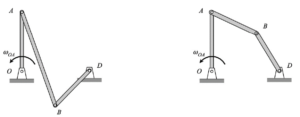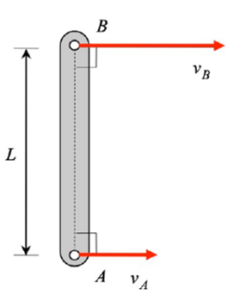# Homework H3.E - Fa22Ask your questions here. You can also answer questions of others. You can learn from helping others.

DISCUSSION and HINTS

Bar OA has two components of rotation:

• One component of Ω about the fixed J-axis.
• The second component of θ_dot about the moving k-axis.

Write out the angular velocity vector ω in terms of the two components described above.

Take a time derivative of ω to get the angular acceleration α of the bar. When taking this derivative, you will need to find the time derivative of the unit vector k. How do you do this? Read back over Section 3.2 of the lecture book. There you will see: k_dot = ω x k, where ω is the total angular velocity vector of bar OA that you found above.

# Homework H3.F - Fa22Any questions??

# Homework H3.C - Fa22Any questions??

Discussion:

Let's first take a look at the motion of point D. This motion of D is shown in the simulation results below.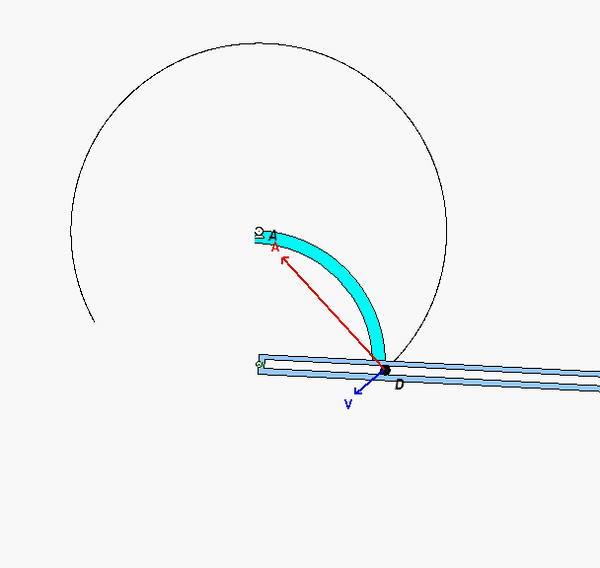The motion of D is circular, with the center of the path located at point A. This is expected since link AD is pinned to ground at point A.

Now, let's attach an observer to link BE. Keep in mind that this observer is unaware that they are moving. The motion that this observer sees is straight, with this straight path aligned with the slot cut into link BE. You can see this is the following animation shown from the perspective of the observer attached to link BE.# Homework H3.D - Fa22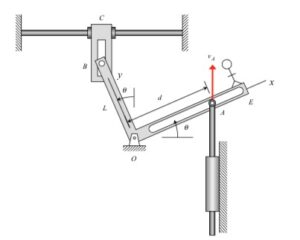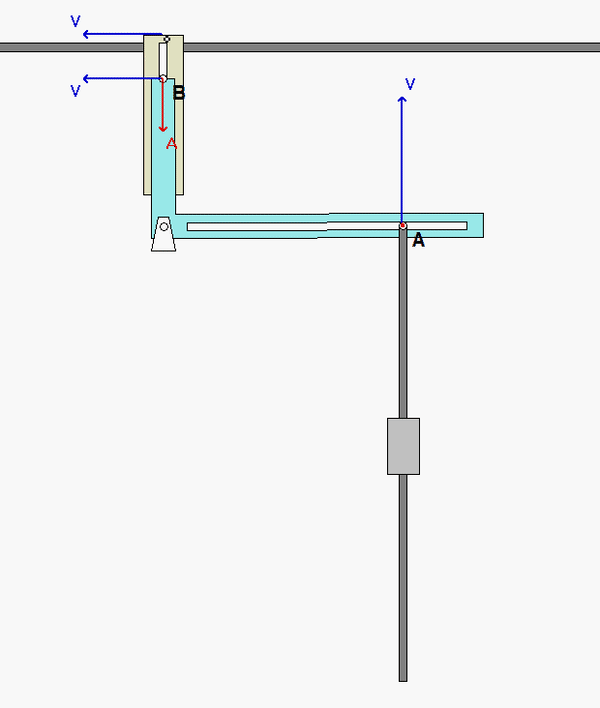# Homework H3.A - Fa22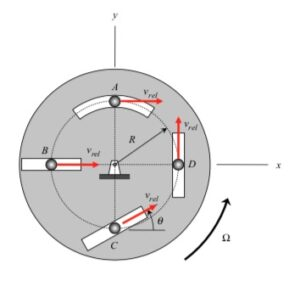Any questions??

# Homework H3.B - Fa22Any questions??

Discussion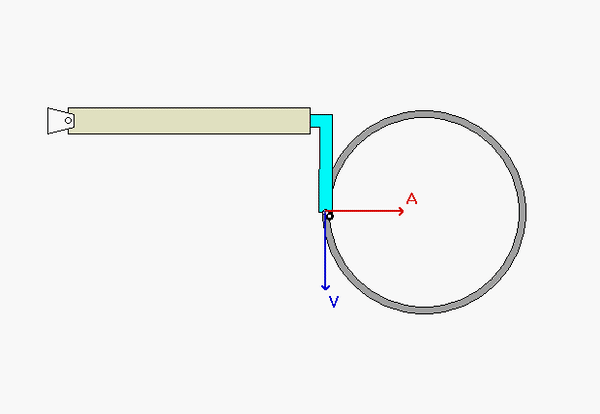Suppose that you decide to use the following velocity and acceleration equations:
vA = vO + (vA/O)rel + ω x rA/O
aA = aO + (aA/O)rel + α x rA/O + 2ω x (vA/O)rel + ω x (ω x rA/O)

using an observer that is attached to link OB. With this choice, the angular velocity and acceleration of the observer are those of link OB:
ω  = ωOB k
α = α
OBk

Now to the question of what motion does the observer see for point A. The observer see A moving back and forth along the x-axis, where the x-axis is attached to OB:
(vA/O)rel = b_dot i
(aA/O)rel = b_ddot i

Note that A is traveling on a circular path of radius R at a constant speed vA. What does this say about the velocity and acceleration vectors for A and B,  vand aAat this instant? Watch the animation above to confirm your response.

# Homework H2.I - Fa22

NOTE: At time marker 13:58 on the solution video for this problem, a sign error was made in writing down the y-component of the position vector rE/D. Below shows the needed subsequent corrections in the solution due to this sign error.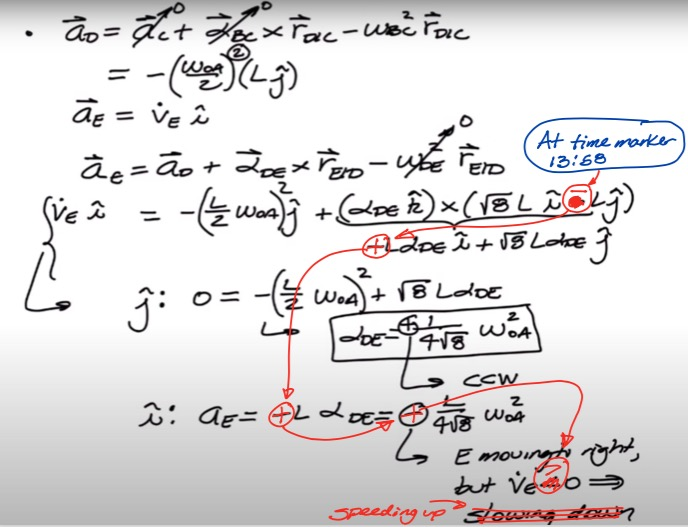We encourage you to interact with your colleagues here in conversations about this homework problem.

Before starting this problem, make note of the type of motion for each component in the mechanism:

• Links OA and BC are in pure rotation about their centers of rotation O and C, respectively. From this, we know that the paths of points A, B and C are circular, as seen in the animation below.
• Block E is in pure translation.
• Links AB and DE have both translational and rotation components of motion.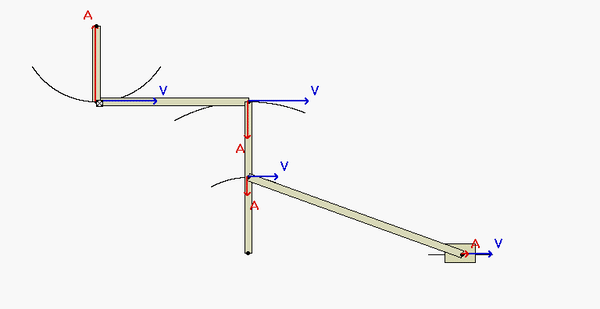Question: What are the locations of the instant centers (ICs) of AB and DE at this instant? Reflect back on the observations above in answering this. What do these locations say about the angular velocities of AB and DE at this position?HINTS:
Once you have found the angular velocities for all of the links, you can then tackle the acceleration analysis.

• For finding the angular acceleration of links AB and BC, use the following rigid body acceleration equations:
aA = aO + αOA x rA/O - ωOA2rA/O
aB = aC + αBC x rB/C - ωBC2rB/C
aB = aA + αAB x rB/A - ωAB2rB/A
This will give you the equations that you need to solve for the desired angular accelerations.
• Repeat the above for link DE to determine its angular acceleration:
aD = aC + αBC x rD/C - ωBC2rD/C
aE = aD + αDE x rE/O - ωDE2rE/O

# Homework H2.J - Fa22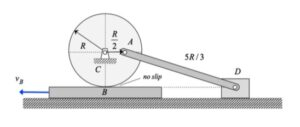Any questions??

Before starting this problem, make note of the type of motion for each component in the mechanism:

• Block B is in pure translation, and moves to the left with a constant speed of vB.
• The disk is pinned to ground at its center C and moves in pure rotation; that is, C is the center of rotation of the disk. Because of this, the velocity of A is in the vertical direction.
• Slider D is in pure translation, and, at the instant of interest, D moves in the same direction as does Block B.
• Link AB has both translational and rotation components of motion.

Question: Where is the location of the instant center (IC) of AD at this instant? Reflect back on the observations above in answering this. You can use the location of the IC for AB to either find the angular velocity of AD, or to check your answer found from vector analysis.HINTS:

• Use the rigid body kinematics equations relating the motion of the contact point on the disk (call that point E) to the center C of the disk to find the angular velocity and angular acceleration of the disk:
vE = vC + ωdisk x rE/C
aE = aC + αdisk x rE/C - ωdisk2 rE/C
• Use the rigid body kinematics equations relating the motion of points A and B to find the angular velocity and angular acceleration of the link AD:
vD = vA + ωAD x rD/A
aD = aA + αAD x rE/C - ωAD2 rD/A

Shown below is a freeze-frame capture of the animation of the motion for this mechanism at the position of interest (when link AC is horizontal). Note that the velocity of A is to the RIGHT at this position. The direction of motion quickly changes to the LEFT at the point where D becomes the IC of AD, as it should.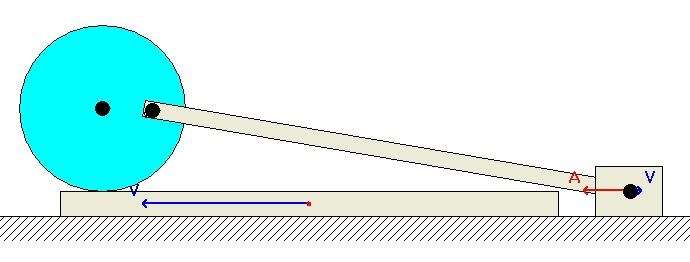# Homework H2.G - Fa22Discussion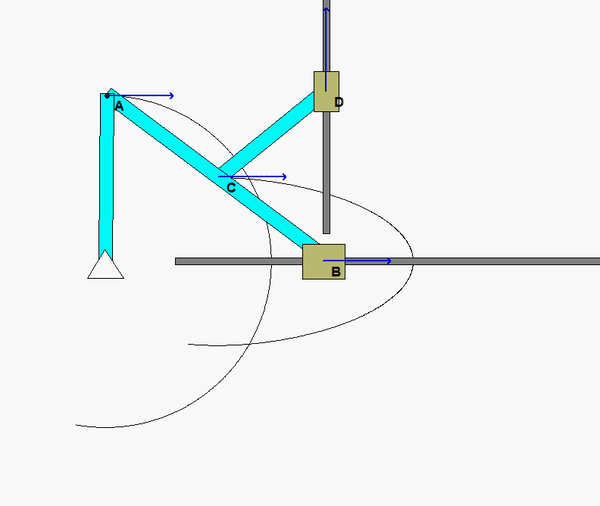The animation above shows the motion of the mechanism over a range of input angles of link OA. For a given position, envision the location of the instant centers (ICs) for links AB and CD. Do the directions and magnitudes for the velocities of points B, C and D agree with the location of these ICs?

Shown below if a freeze-frame of the mechanism motion at the position for which you are asked to do analysis. From this figure, where are the two ICs for links CD and BC? In particular, how does the position of the IC for AB relate to the relative sizes of the speeds of points A, C and B? What is the angular velocity of link AB at this position?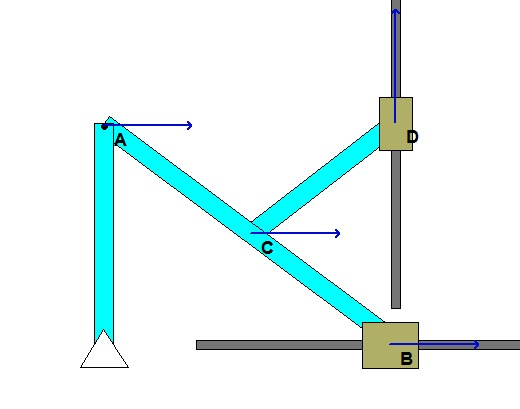# Homework H2.H - Fa22